# What is intensity?

Sound waves are characterized by compression and expansion of the medium as sound energy moves through it. This represents the pressure component of sound. At the same time, there is also back and forth motion of the particles making up the medium (particle motion).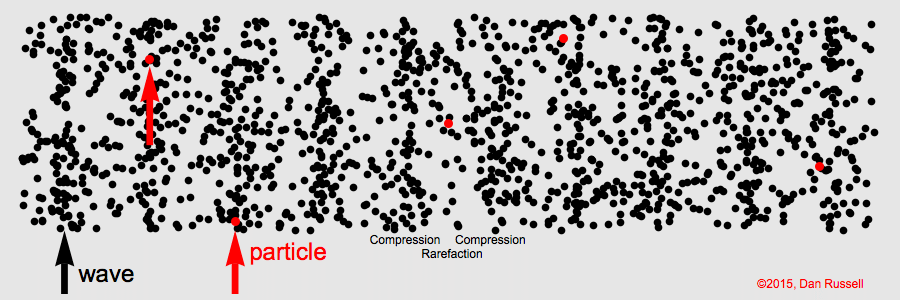Sound is a longitudinal wave. Animation credit: Dan Russell, Grad. Prog. Acoustics, Penn State, http://www.acs.psu.edu/drussell/Demos/waves/wavemotion.html

Particle motion is described by displacement, velocity, and acceleration.

Sound intensity depends on both particle velocity ($\overrightarrow{u}$ ) and pressure (p). The arrow over the symbol for particle velocity indicates that velocity is a vector quantity; it has both a magnitude and direction. The motion of the particles in a sound wave is aligned with the direction in which the wave is traveling. Pressure, on the other hand, does not have a direction associated with it.

The intensity$\overrightarrow{I}$ ) of a sound wave is defined as the amount of energy transmitted through a unit area per unit time in the direction that the sound wave is traveling. It is given by the product of the pressure and velocity of the sound wave:$\overrightarrow{I} = p \overrightarrow{u}$

The particle velocity and pressure in a sound wave are related. For a plane wave the relationship is: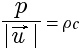The symbol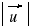indicates the magnitude of the particle velocity, without considering the direction. This ratio depends only on the density (ρ ) and sound speed (c ) of the medium through which the sound wave is traveling. It is called the characteristic acoustic impedance of the medium: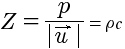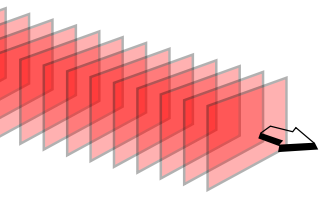Diagram of a plane wave. The red rectangles represent wavefronts of a plane wave traveling in three-dimensional (3-D) space.

One role of the middle ear in terrestrial mammals is to overcome the mismatch between the acoustic impedances of air and the fluid in the inner ear. (People do not hear well underwater because the human ear is designed to match impedances between air and the inner ear, not between water and the inner ear.)

Using the previous equation, particle velocity for a plane wave can be written as a function of pressure, density, and sound speed: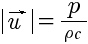The intensity of a sound therefore depends not only on the pressure (p), but also on the density (ρ ) and sound speed (c ) of the medium through which the sound is traveling: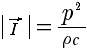Sounds in water and sounds in air that have the same pressures have very different intensities because the density of water is much greater than the density of air, and because the speed of sound in water is much greater than the speed of sound in air. For the same pressure, higher density and higher sound speed both give a lower intensity. The result is that sound waves with the same intensities in water and air when measured in watts per square meter have sound levels that differ by 61.5 dB.

The most common device for measuring underwater sound is the hydrophone. Hydrophones measure the pressure (amplitude) of a sound wave, rather than its intensity. Hydrophones are not sensitive to particle velocity. The sound intensity level is therefore calculated from the measured sound pressure, using the relationship between the magnitude of the intensity and pressure. (for more detail, see: Introduction to Decibels)

Geophones measure particle velocity, but are more commonly used to measure seismic waves than underwater sound waves. Vector sensors measure particle velocity or acceleration together with pressure to provide direct measurements of intensity, including the direction in which the sound wave is traveling. Vector sensors are used in DIFAR (Directional Frequency Analysis and Recording) sonobuoys, for example.